Tutorial for Diameter Dimensions¶

Note

Ezdxf does not consider all DIMSTYLE variables, so the rendering results are different from CAD applications.

This is a repetition of the radius tutorial, just with diameter dimensions.

import ezdxf

# setup=True setups the default dimension styles
doc = ezdxf.new("R2010", setup=True)

msp = doc.modelspace()  # add new dimension entities to the modelspace
# add default diameter dimension, measurement text is located outside
center=(0, 0),
angle=45,
)
dim.render()
doc.saveas("diameter_dimension.dxf")

The example above creates a 45 degrees slanted diameter Dimension entity, the default dimension style “EZ_RADIUS” (same as for radius dimensions) is defined as 1 drawing unit = 1m, drawing scale = 1:100 and the length factor = 100, which creates a measurement text in cm, the default location for the measurement text is outside of the circle.

The center point defines the the center of the circle but there doesn’t have to exist a circle entity, radius defines the circle radius and angle defines the slope of the dimension line, it is also possible to define the circle by a measurement point mpoint on the circle.

The return value dim is not a dimension entity, instead a DimStyleOverride object is returned, the dimension entity is stored as dim.dimension.

Placing Measurement Text¶

There are different predefined DIMSTYLES to achieve various text placing locations.

The basic DIMSTYLE “EZ_RADIUS” settings are:

• 1 drawing unit = 1m
• scale 1:100
• the length factor dimlfac = 100, which creates a measurement text in cm.
• uses a closed filled arrow, arrow size dimasz = 0.25

Note

Not all possibles features of DIMSTYLE are supported by the ezdxf rendering procedure and especially for the diameter dimension there are less features implemented than for the linear dimension because of the lack of good documentation.

Default Text Locations Outside¶

“EZ_RADIUS” default settings for to place text outside:

 tmove 1 = add a leader when dimension text is moved, this is the best setting for text outside to preserve the appearance of the DIMENSION entity, if editing afterwards in a CAD application. dimtad 1 = place the text vertical above the dimension line
center=(0, 0),
angle=45,
)
dim.render()  # always required, but not shown in the following examples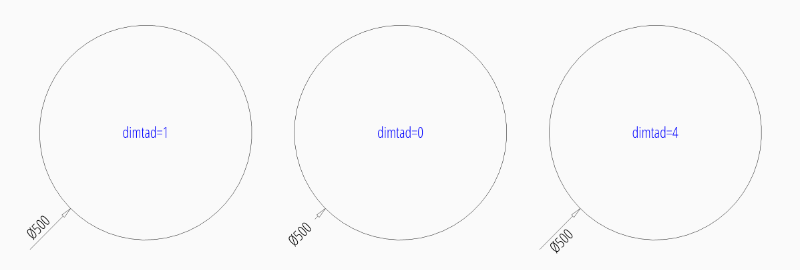To force text outside horizontal set dimtoh to 1:

center=(0, 0),
angle=45,
override={"dimtoh": 1}
)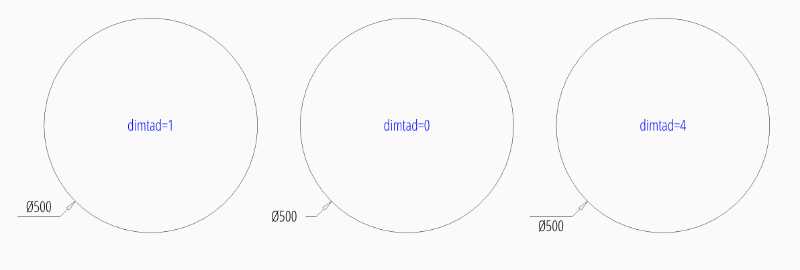Default Text Locations Inside¶

DIMSTYLE “EZ_RADIUS_INSIDE” can be used to place the dimension text inside the circle at a default location.

The basic DIMSTYLE settings are:

• 1 drawing unit = 1m
• scale 1:100, length_factor is 100 which creates
• the length factor dimlfac = 100, which creates a measurement text in cm.
• uses a closed filled arrow, arrow size dimasz = 0.25

Advanced “EZ_RADIUS_INSIDE” settings to place (force) the text inside of the circle:

 tmove 0 = moves the dimension line with dimension text, this is the best setting for text inside to preserve the appearance of the DIMENSION entity, if editing afterwards in a CAD application. dimtix 1 = force text inside dimatfit 0 = force text inside, required by BricsCAD and AutoCAD dimtad 0 = center text vertical, BricsCAD and AutoCAD always create a vertical centered text, ezdxf let you choose the vertical placement (above, below, center), but editing the DIMENSION in BricsCAD or AutoCAD will reset text to center placement.
center=(0, 0),
angle=45,
)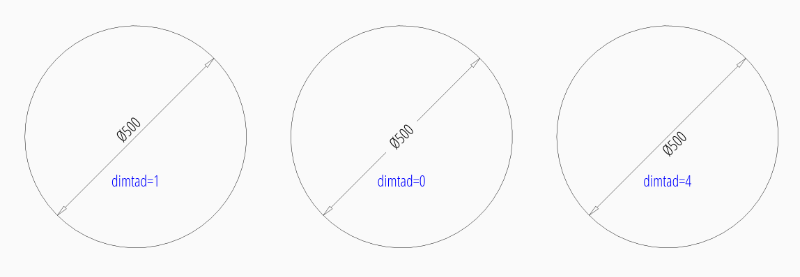To force text inside horizontal set dimtih to 1:

center=(0, 0),
angle=45,
override={"dimtih": 1}
)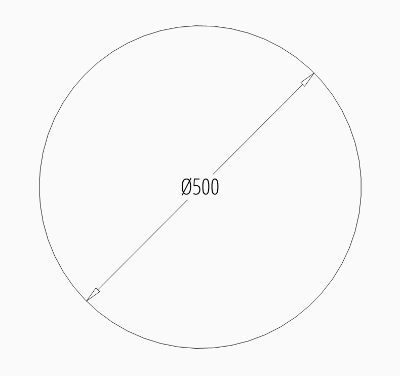User Defined Text Locations¶

Beside the default location it is always possible to override the text location by a user defined location. This location also determines the angle of the dimension line and overrides the argument angle. For user defined locations it is not necessary to force text inside (dimtix=1), because the location of the text is explicit given, therefore the DIMSTYLE “EZ_RADIUS” can be used for all this examples.

User defined location outside of the circle:

center=(0, 0),
location=(4, 4),
)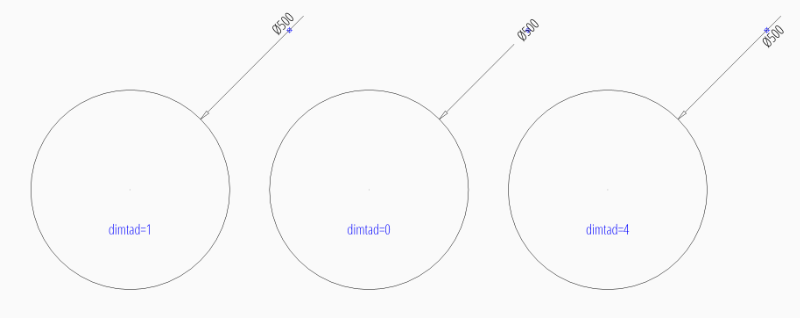User defined location outside of the circle and forced horizontal text:

center=(0, 0),
location=(4, 4),
override={"dimtoh": 1}
)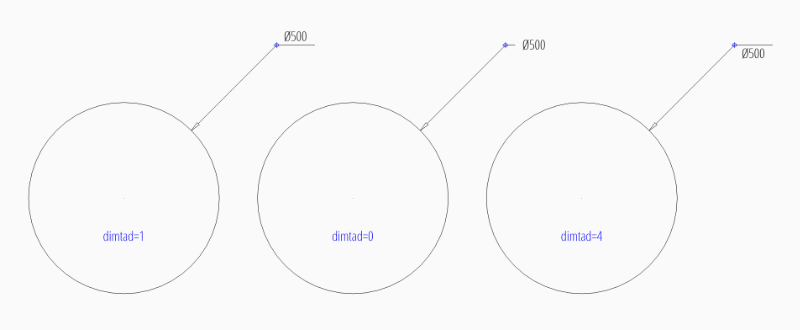User defined location inside of the circle:

center=(0, 0),
location=(1, 1),
)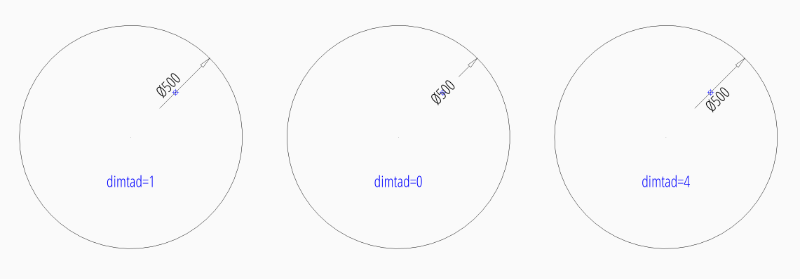User defined location inside of the circle and forced horizontal text:

center=(0, 0),
location=(1, 1),
override={"dimtih": 1},
)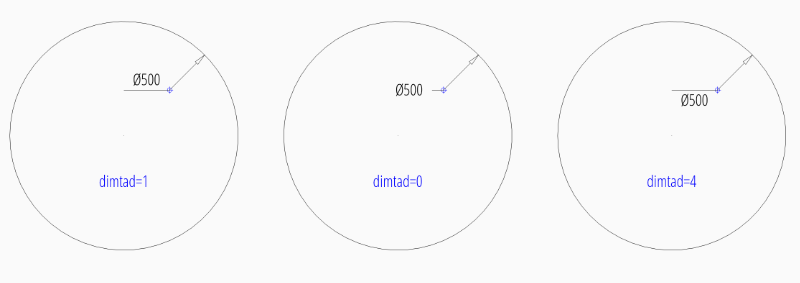Center Mark/Lines¶

See Radius Dimension Tutorial: Center Mark/Lines

Overriding Measurement Text¶

See Linear Dimension Tutorial: Overriding Measurement Text

Measurement Text Formatting and Styling¶

See Linear Dimension Tutorial: Measurement Text Formatting and Styling